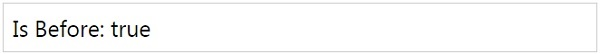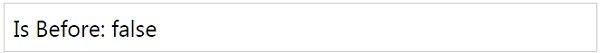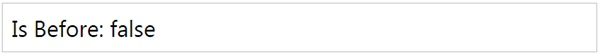# MomentJS - Is Before

This method checks if the given moment is before another moment. It returns true or false.

## Syntax

```moment().isBefore(Moment|String|Number|Date|Array);
moment().isBefore(Moment|String|Number|Date|Array, String);
```

## Example

```var isbefore = moment().isBefore('2020-10-21');
```

## OutputNote that the date used inside isBefore is greater than the current date, so we are getting true as the output.

## Example

```var isbefore = moment([2015, 10, 01]).isBefore([2000, 10, 21]);
```

## OutputWith isBefore, we can compare dates from one moment to another. We can also specify the units with isBefore. The units supported are year, month, week, day, hour, minute and second.

## Example

Let us consider a working example with units passed to isBefore();

```var isbefore = moment([2015, 10, 01]).isBefore([2010, 10, 21], 'year');
```

## Outputmomentjs_date_queries.htm